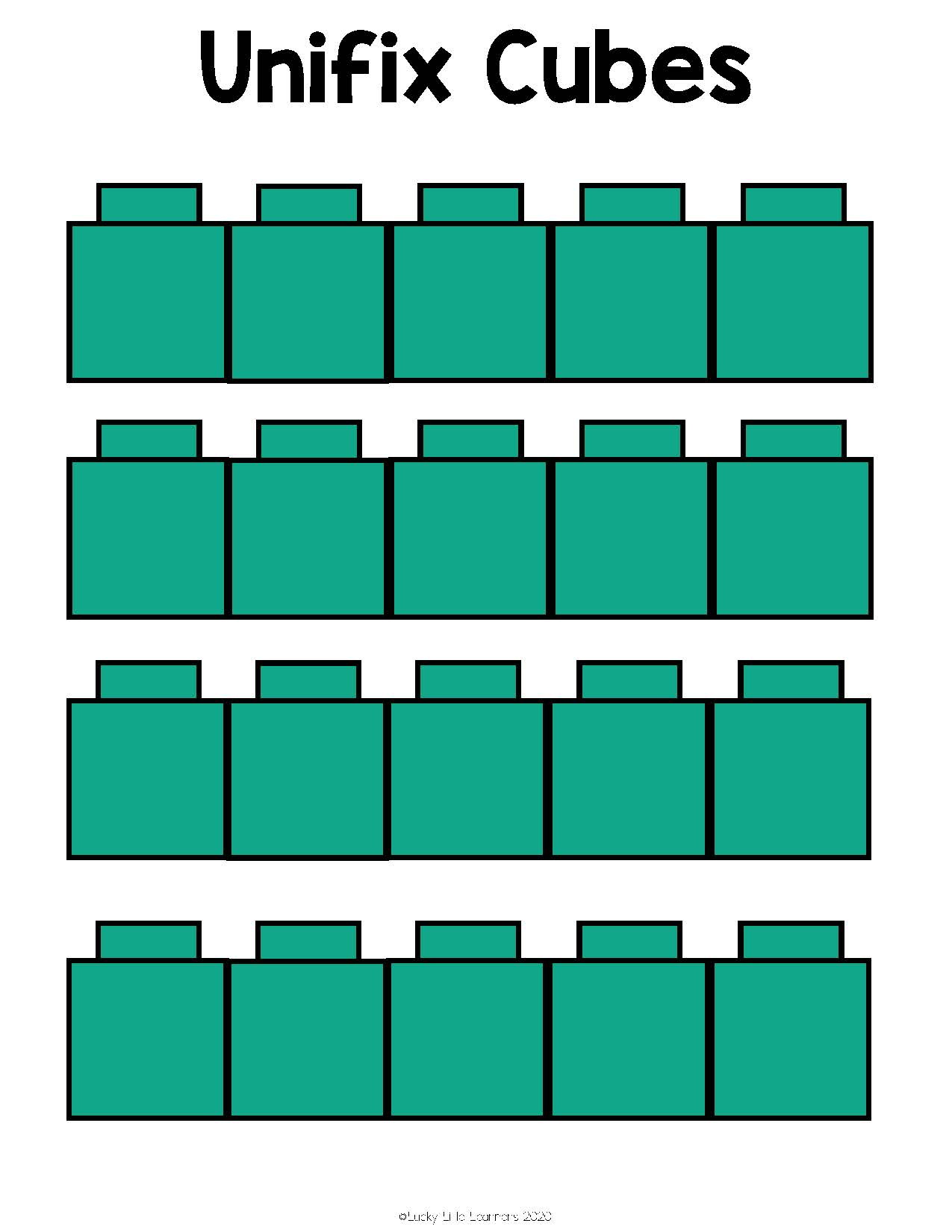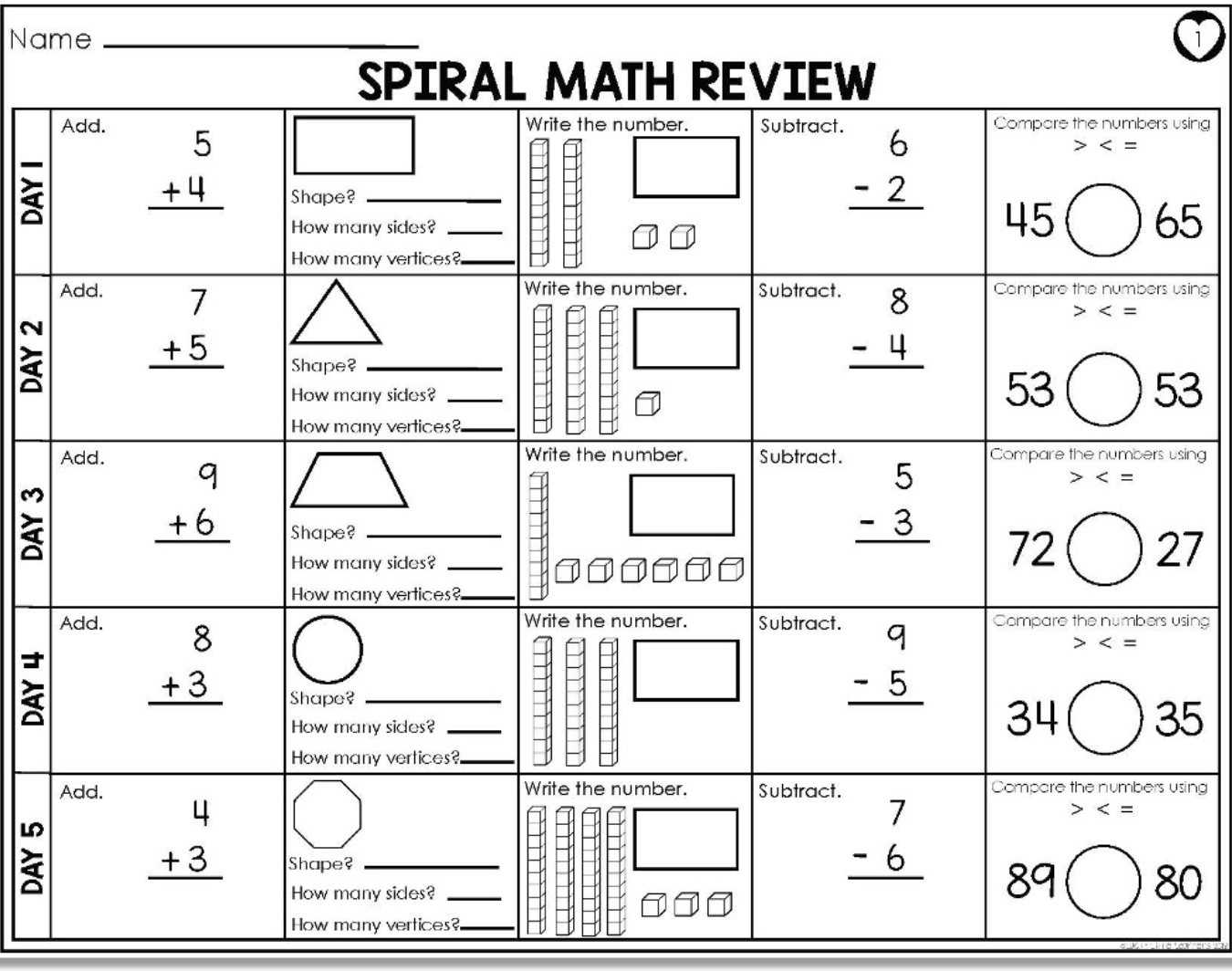Home » Blog » Operations » Word Problems » How to Teach Math Word Problems in 2nd Grade

# How to Teach Math Word Problems in 2nd Grade

Written by: Mary Kate Bolinder

Your students have mastered their math facts. They understand basic operations. Now it’s time to tackle some word problems! Read on to discover strategies and resources to help teach word problems to 2nd grade learners.

## Why is it important to teach word problems?

Why is it important to teach word problems? Answer: it’s not just because it’s a grade level standard! Word problems allow students to demonstrate a full understanding of the math skills and operations they have learned. Word problems also allow students to use logic, critical thinking, exercise a step-by-step approach, and identify essential information. All of these skills listed are not only important math skills, but also important life skills!

## 5 Word Problems Strategies for 2nd Grade

There are many different strategies to teach students to solve 2nd grade math word problems. Try one, some, or all of the strategies listed to reach learners of all levels and abilities.

### 1. Identify Key Words for Each Operation

In every 2nd grade math word problem, there is an important keyword. This keyword will unlock the answer by telling you what operation must be used to correctly solve the problem. For students to be able to identify the correct operation, they need to recognize and understand the word problem keywords for addition and subtraction.

Common keywords for addition word problems:

• in all
• altogether
• sum
• total
• increased by
• plus
• combined

Common keywords for subtraction word problems:

• difference
• less than
• take away
• minus
• fewer
• left over/left
• remain
• minus
• how many more

Practice reading word problems aloud together. Can your students spot the keyword in each problem?

### 2. CUBES Math Strategy for Word Problems

The CUBES strategy is a helpful way to solve 2nd grade word problems. Use this acronym to help you remember the steps needed to solve a word problem:

C: Circle the numbers

U: Underline the question

B: Box the keywords

E: Eliminate the extra information

S: Solve and check the problem

By using the CUBES strategy, the student is able to break down the word problem into small, manageable parts. This blog post about the CUBES strategy explains everything you need to know about using this strategy with 2nd grade learners. You can download the CUBES math strategy chart FREEBIE here.

### 3. Draw a Picture to Solve Math Word Problems

A word problem is a math story. Visual learners may benefit from drawing a picture to solve a math word problem. No need to be an artist! Students can draw simple shapes to represent the items in the word problem.

Addition Word Problem Example: There are 21 purple butterflies and 8 pink butterflies in the garden. How many butterflies are there in all?

To solve this problem, a student would draw 21 purple butterflies (or dots/symbols to represent a butterfly), and 8 pink butterflies. Then count all the butterflies to get the answer.

Subtraction Word Problem Example: There are 21 butterflies in the garden. 8 fly away. How many butterflies are left?

To solve this word problem, students would again draw 21 butterflies. To show subtraction, put an X over 8 butterflies. How many remain?

This video does a great job of showing how to use pictures to solve a math word problem:

### 4. Use objects to solve a word problem

Use math manipulatives to solve word problems! 2nd grade learners will benefit from this visual, hands-on learning aid! Some math manipulatives that can be used to solve word problems include unifix cubes, base ten blocks, counters, erasers, tangrams, popsicle sticks – anything tactile that will help students visualize the problem will do! Also, here’s a list of some of our favorite math manipulatives. A variety of manipulatives can be printed from the Lucky Little Toolkit.### 5. Write a number sentence

You’ve found the numbers. You know what operation to use. Now it’s time to show that work by writing a number sentence! Solve to find your answer. Need more practice with word problems? Check out the Spiral Math Review Freebie for leveled review!# 1 Comment

1.I love the You Tube video that explains the strategy, however, the child’s name in the problem is Jayden and you say “Troy” has five more after solving the problem. Just a tad confusing for kids.